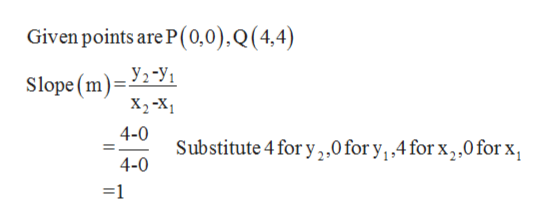# Use the point slope formula to write the equation of the line passing through the two given points. Write each answer in slope-intercept form. P(0,0), Q(4,4)

Question
71 views

Use the point slope formula to write the equation of the line passing through the two given points. Write each answer in slope-intercept form.

P(0,0), Q(4,4)

check_circle

Step 1

Find the equation of the line passing through the two given points. Write each answer in slope-intercept form.

P(0,0), Q(4,4)

Step 2

First find the slope...help_outlineImage TranscriptioncloseGiven points are P(0,0), Q (4,4) Уз-У, Slope (m X, -х, 4-0 Substitute 4 for y2,0 for y.4 for x2,0 for x 4-0 =1 fullscreen

### Want to see the full answer?

See Solution

#### Want to see this answer and more?

Solutions are written by subject experts who are available 24/7. Questions are typically answered within 1 hour.*

See Solution
*Response times may vary by subject and question.
Tagged in

### Other